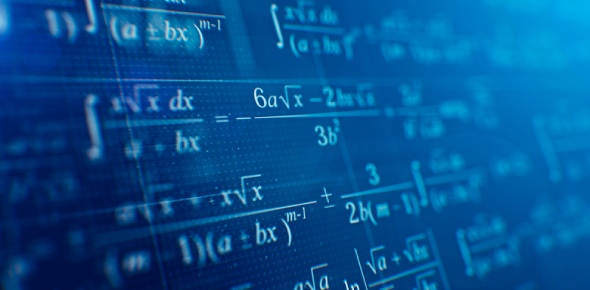# Quiz: Mathematics Ss1 First Term #2

20 Questions | Total Attempts: 2295SettingsDo you think mathematics is complicated, or is it fun for you? If you are good at it, you will likely enjoy solving complex equations and simplifying tables. For this quiz, you should know things such as how to solve for equalities and calculate the length of something at an angle at the center of a circle of radius. This quiz will demonstrate your knowledge of mathematics.

• 1.
Solve the following inequality: 28-5x < x-4.
• A.

X>6

• B.

X<5.33

• C.

X>5.33

• D.

X>8

• 2.
Which of the following is NOT a measure of central tendencies?
• A.

Median

• B.

Mean deviation

• C.

Decile

• D.

Mode

• 3.
Simplify without using tables: 13/4 + 31/5 X 12/3+ 1/12
• A.

13/4

• B.

21/4

• C.

53/6

• D.

Option 4

• 4.
What is the length of an arc, which subtends an angle 600 at the centre of a circle of radius 0.5m?
• A.

4.21

• B.

2.31

• C.

1.13

• D.

0.52

• 5.
Evaluate 7 - 35 = (mod 8).
• A.

-6

• B.

-4

• C.

2

• D.

4

• 6.
Esther was facing S20°W. She turned 90° in the clockwise direction. What direction is she facing now?
• A.

S70°E

• B.

N70°W

• C.

N70°E

• D.

S70°W

• 7.
What is the area of a circle whose radius is 7cm. (Take π = 22/7).
• A.

54cm2

• B.

84cm2

• C.

154cm2

• D.

184cm2

• 8.
Find the circumference of a circle whose diameter is 10cm. (Take π = 22/7).
• A.

31.42 cm

• B.

21.23 cm

• C.

14.16 cm

• D.

9.13 cm

• 9.
Write 123.6 in standard form.
• A.

1.236 X 10-1

• B.

1.236 X 101

• C.

1.236 X 102

• D.

1.236 X 103

• 10.
Write in a combined inequality the following: x < 3, x > -2.
• A.

-2 < x < 3

• B.

-2 < x > 3

• C.

X > 3 < 2

• D.

-2 < x - 3

• 11.
If y varies inversely as the square of x, and y = 11/4 when x = 4, find the value of y when x = 1/2
• A.

-1

• B.

5

• C.

10

• D.

15

• 12.
Find the three lowest values of x, given that x is the integer: 18 + 5x > 1.
• A.

-3,-4,-5

• B.

0,1,2

• C.

-1,-2,-3

• D.

-2,-1,0

• 13.
Given C=(5(F-32))/9, find F when C = 35.
• A.

15

• B.

37

• C.

69

• D.

95

• 14.
Find the equation of the lines passing through the following pair of points: A (2 ,3) and B (3 ,9).
• A.

Y = 6x-9

• B.

Y = 5x-9

• C.

Y = 6x-8

• D.

Y = 5x-7

• 15.
Use logarithm tables to evaluate the following: 608  *  4.85 ÷ 276.
• A.

10.69

• B.

9.69

• C.

8.96

• D.

1.06

• 16.
Factorise 2x2 + 9x + 7 (A) (x+1)(2x+1) (B) (3x-1)(2x+1) (C) (x+1)(2x+7) (D) (x+1)(3x+7)
• A.

Option 1

• B.

Option 2

• C.

Option 3

• D.

Option 4

• 17.
Three times a certain number added to 14 is the same as when two times the number is subtracted from 6. What is the number?                  (A)     8/5        (B)    -8/5        (C) -4              (D) -8
• A.

Option 1

• B.

Option 2

• C.

Option 3

• D.

Option 4

• 18.
Simplify √147 - √75 + √12, leaving your answer in surd form. (A) 14√3 (B) 3√3 (C) 4√3 (D) 28 √3
• A.

Option 1

• B.

Option 2

• C.

Option 3

• D.

Option 4

• 19.
The operation * on the set Q of rational numbers is defined by p*q=(p+q-3pq)/2, p,q∈Q. Determine -2*3. (A) 17 (B) -8.5 (C) 8.5 (D) -9.2
• A.

Option 1

• B.

Option 2

• C.

Option 3

• D.

Option 4

• 20.
Which one do you like? Express 8.75/0.025 in standard form (A) 3.5 x 103 (B) 3.5 x 102 (C) 3.5 x 101 (D) 3.5 x 102
• A.

Option 1

• B.

Option 2

• C.

Option 3

• D.

Option 4

Related TopicsBack to top